# Modeling, Forecasting and Simulating Commodity Prices through Term Structure Estimation using Kalman Filtering: The R Package ‘NFCP’

library(NFCP)

# Set seed for reproducibility:
set.seed(412)

# 1. Introduction

‘NFCP’ (N-Factor Commodity Pricing) provides a framework for the modeling, parameter estimation, probabilistic forecasting, European and American options on futures contracts valuation and simulation of commodity prices through state space and Monte Carlo methods, risk-neutral valuation and Kalman filtering. Commodity pricing models are systems of stochastic differential equations that are utilized for the valuation and hedging of commodity contingent claims (i.e. derivative products on the commodity) and other commodity related investments. Parameters of commodity pricing models are estimated through maximum likelihood estimation (MLE), using available term structure futures data of a commodity. Commodity pricing models that capture market dynamics are of great importance to commodity market participants in order to exercise sound investment and risk-management strategies. The N-factor commodity pricing model framework was first presented in the work of Cortazar and Naranjo (2006) titled “An N-Factor Gaussian Model of Oil Futures Prices”.

The purpose of this vignette is to provide worked examples of the estimation and analysis of commodity pricing models using the N-factor framework of the ‘NFCP’ package. Examples presented within this vignette, as well as throughout the documentation of the ‘NFCP’ package, replicate the prolific work of Schwartz and Smith (2000) titled: “Short-Term Variations and Long-Term Dynamics in Commodity Prices”. The two-factor “Short-Term/Long-Term” model presented within this study is replicated, with the primary figures and results replicated as well as discussion on how to interpret and analyze N-factor models. The remainder of this vignette is structured as follows. Section 2 provides a background of modeling commodity prices through term structure estimation. Section 3 introduces the N-factor stochastic modeling framework. Section 4 provides worked examples on the estimation and analysis of commodity pricing models, using the crude oil data first presented within the work of Schwartz and Smith (2000), replicating many of the results of this study. Section 5 provides a discussion of the N-factor commodity pricing model, the ‘NFCP’ package and concludes.

# 2. Term Structure Estimation

Modeling commodity prices is typically performed through term structure estimation, as futures markets provide valuable information regarding expectations of future price movements and other dynamics. Parameter estimation of commodity pricing models involves expressing the futures curve in terms of unobserved factors and deriving analytic expressions for futures prices under no-arbitrage conditions. Term structure estimation of commodity pricing models was popularized within the works of Gibson and Schwartz (1990), Schwartz (1997, 1998) and Schwartz and Smith (2000). These commodity pricing models make differing assumptions regarding the innovation, mean-reverting behavior, consideration of the cost-of-carry, and the number of correlated factors to explain the term structure of commodities. These commodity pricing models (or their affine transformation equivalents, see Cortazar and Naranjo (2006)) were subsequently unified under the N-factor framework of Cortazar and Naranjo (2006), expressing the log of the commodity spot price processes as the sum of N correlated factors. ‘NFCP’ considers commodity pricing models under this N-factor framework.

Kalman filtering is the process of obtaining unreliable, but measurable, observations and estimating a time series of unobservable measurements in return, with an allowable degree of measurement error. Kalman filtering is used to estimate parameters of commodity pricing models through MLE (Gibson and Schwartz (1990), Schwartz (1997, 1998), Schwartz and Smith (2000), Cortazar and Naranjo (2006), Aspinall et al. (2020). Parameter estimation of N-factor models features many unknown parameters, a large number of observations and an objective function (the log-likelihood) that is discontinuous and nonlinear, with multiple local optima. The complexity of parameter estimation increases substantially according to the number of factors in the model. The number of unknown parameters of an N-factor model (without considering the measurement error of futures contracts) is equal to: $$0.5 (N^2 + 5N)$$. MLE is performed using genetic algorithms through the ‘genoud’ function of the ‘rgenoud’ package to maximize the likelihood of obtaining MLE values.

# 3. The N-Factor Model

The N-factor commodity pricing model was first presented in the work of Cortazar and Naranjo (2006, equations 1-3). Following the work of Cortazar and Naranjo (2006), the N-factor framework describes the spot price process of a commodity as the correlated sum of $$N$$ state variables $$x_{t}$$.

$log(S_{t}) = \begin{cases} \sum_{i=1}^N x_{i,t} &\text{GBM = T} \\ E+\sum_{i=1}^N x_{i,t} &\text{GBM = F} \\ \end{cases}$

where state variables (i.e. factors of the spot price) follow correlated mean-reverting (i.e. Ornstein-Uhlenbeck) processes, with the first state variable further able to be considered to follow a random walk (i.e. Brownian motion process) to induce a unit root in the spot price process when logical argument $$GBM$$ is true. The valuation of derivative products and commodity-related investments requires the “risk-neutral” version of the model. Here (and below) asterisks are used to denote the risk-neutral versions of variables and processes rather than the “true” process. Under the assumption of risk-neutrality the state variables follow the process:

$dx_{1,t} = \begin{cases} \mu^*dt + \sigma_{1} dw_{1}t &\text{GBM = T} \\ - (\lambda_{1} + \kappa_{1}x_{1,t})dt + \sigma_{1} dw_{1}t &\text{GBM = F} \\ \end{cases}$

$dx_{i,t} =_{i\neq 1} - (\lambda_{i} + \kappa_{i}x_{i,t})dt + \sigma_{i} dw_{i}t$

Where: $E(w_{i})E(w_{j}) = \rho_{i,j}$

Additionally, deterministic seasonality can be considered within the ‘NFCP’ package through the sum of trigonometric sin() and cos() functions:

$season(t) = \sum_{i=1}(season_{i,1}.\cos(2.i.\pi) + season_{i,2}.\sin(2.i.\pi)$ The addition of deterministic seasonality is compatible with all functions of the ‘NFCP’ package.

The following constants are defined as:

$$\mu$$: long-term growth rate

$$E$$: Constant equilibrium level

$$\kappa_{i}$$: Reversion rate of state variable $$i$$

$$\sigma_{i}$$: the instantaneous volatility of state variable $$i$$

$$\rho_{i,j} \in [-1,1]$$: the instantaneous correlation between state variables $$i$$ and $$j$$

The risk-neutral variables are defined as:

$$\mu^*=\mu-\lambda_1$$: Long-term risk-neutral growth rate

$$\lambda_{i}$$: Risk premium of state variable $$i$$

Under the assumption of risk-neutrality, state variables with mean-reverting behaviour revert towards the equilibrium value $$- \frac{\lambda_{i}}{\kappa_{i}}$$ at a rate of $$\kappa_{i}$$ with a respective half-life of $$\frac{log(2)}{\kappa_{i}}$$.

The N-factor framework is a linear Gaussian framework. This means that the state variables under the risk-neutral process are jointly distributed with mean vector and covariance matrix:

$E^*[x_i(t)] = \begin{cases} \ x_i(0) + \mu^* t &i=1 \text{ and GBM=T} \\ \ x_i(0)e^{-(\kappa_i t)} - \frac{(1-e^{-\kappa_it})\lambda_i}{\kappa_i} &\text{otherwise} \\ \end{cases}$ and:

$Cov^*_{i,j}(x_{i,t},x_{j,t}) = Cov_{i,j}(x_{i,t},x_{j,t}) = \begin{cases} \sigma_1^2(t) &i=1,j=1 \text{ and GBM = T} \\ \sigma_i\sigma_j\rho_{i,j}\frac{1-e^{-(\kappa_i+\kappa_j)t}}{\kappa_i+\kappa_j} &\text{otherwise} \\ \end{cases}$

Filtering the N-factor model through the Kalman filter to estimate the optimal one-step-ahead predictions of state variables is described in ‘help(NFCP_Kalman_filter)’.

# 4. Using the ‘NFCP’ package:

The following section provides worked examples on the functionality of the ‘NFCP’ package, detailing how to estimate, analyse and apply commodity pricing models using the various functions of the package. Major findings, plots and tables from the highly-cited work of Schwartz and Smith (2000) are replicated in this section. A one-factor geometric Brownian motion model with deterministic seasonality is also estimated and interpreted. Measures of robustness and performance of these models are also presented.

The Short-Term/Long-Term model of Schwartz and Smith (2000) is one of the most popular commodity pricing models, and is a special case of the N-factor framework when N=2. The first factor of the Short-Term/Long-Term model follows a Brownian motion. The approximate 1990-1995 crude oil data, as well as estimated parameters and modeling assumptions, used within the original work of Schwartz and Smith (2000) is included within the ‘SS_oil’ list object. See help(SS_oil) for more details.

The constant parameters of the Short-Term/Long-Term model are:

model_parameters_2F <- NFCP_parameters(N_factors = 2,
GBM = TRUE,
initial_states = FALSE,
N_ME = 5)
#>
#> ----------------------------------------------------------------
#> 2 Factors model: first factor is a GBM
#>
#> Risk Neutral SDE:
#>
#> log(s_t) = sum(x_t)
#>
#> Where:
#> d x1_t    = mu_rn * dt  + sigma_1 * dW_1
#> d x2_t    = - (lambda_2 + kappa_2 * x2_t) * dt  + sigma_2 * dW_2
#>
#>  And:
#>
#> E(dW_1 * dW_2) = rho_1_2 * dt
## Print the vector of parameters of the specified commodity pricing model:
print(model_parameters_2F)
#>   "mu"       "mu_rn"    "lambda_2" "kappa_2"  "sigma_1"  "sigma_2"
#>   "rho_1_2"  "ME_1"     "ME_2"     "ME_3"     "ME_4"     "ME_5"

N-factor models utilized throughout the ‘NFCP’ package are generally provided within the arguments of functions as a named vector of parameter values. The argument ‘N_ME’ corresponds to the number of measurement errors to consider during Kalman filtering. In Schwartz and Smith (2000), five futures contracts were observed at each time periods, thus five independent measurement errors were specified. Measurement errors are discussed in greater detail in section 4.3.

## 4.1 Stitching Futures Data

Traditional implementations of the Kalman filter to term structure data (Schwartz, 1997; Schwartz and Smith, 2000, among others) stitched futures contracts together according to their ordering by maturity. This results in a dataset where “for all given dates in the estimation sample, prices for the same set of contracts (with the same maturities) (are observed)” (Cortazar and Naranjo, 2006). Contracts have been stitched within existing literature in order to attain a dataset that has no missing observations and a time homogeneous time-to-maturity of observations - resulting in the Kalman filter quickly approaching a steady state distribution. Contracts are rolled over as the closest contract expires. Aggregating futures data creates information loss due to discrepancies between the actual time-to-maturity of observations and the assumed, time homogeneous time-to-maturity. Information loss also occurs as not all available contracts at a given point in time are observed within the model. Studies that have developed commodity pricing models on stitched data include: Longstaff and Schwartz (1990), Schwartz (1997), Schwartz and Smith (2000), Rennemo (2019), Aspinall et al., (2020).

The approximate stitched data used to estimate commodity pricing models in the work of Schwartz and Smith (2000) is provided within the SS_oil list object. Stitching futures contracts to obtain a complete aggregate panel dataset can be developed through the ‘stitch_contracts’ function.

###Method 1 - Stitch Crude Oil Contracts according to maturity matching:
SS_oil_stitched <- stitch_contracts(futures = SS_oil$contracts, futures_TTM = c(1, 5, 9, 13, 17)/12, maturity_matrix = SS_oil$contract_maturities,
rollover_frequency = 1/12, verbose = TRUE)

##Plot the Stitched Maturities:
matplot(as.Date(rownames(SS_oil_stitched$maturities)), SS_oil_stitched$maturities,
type = 'l', main = "Stitched Contract Maturities",
ylab = "Time To Maturity (Years)", xlab = "Date", col = 1)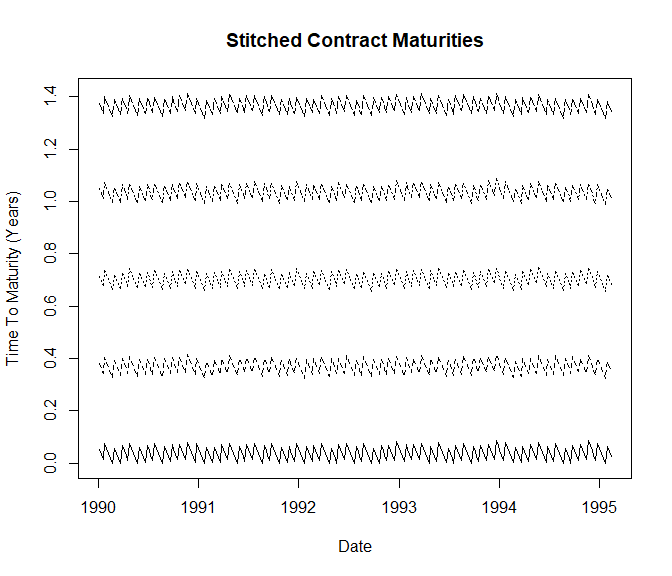The plot above displays the stitching of futures contracts across a five year observation period. This figure is highly similar to Figure 1. of Schwartz (1997), although for a different observation period and different fixed maturities of stitched contracts.

Rather than stitching futures contracts to obtain a complete panel dataset, several other studies have instead used incomplete panel data of all available futures contracts to ensure there is no information loss during MLE (Sørensen, 2002; Cortazar and Schwartz, 2003; Cortazar and Naranjo, 2006). For the Schwartz and Smith (2000) oil contract data, there are an average of 21 available contracts at each observation. Both complete and incomplete panel data is compatible throughout the ‘NFCP’ package.

Whilst stitching data does result in information loss, the increase in relative algorithm efficiency compared to contract data can make this data structure appealing, particularly for estimating commodity pricing models with several state variables. Information loss generally results in lower accuracy in the estimation of longer maturity futures contracts that are discared through the stitching process.

The liquidity of observed futures prices is a key consideration of the term structure data input into the commodity pricing model. One approach was taken by Trolle and Schwartz (2009). From the work of Trolle and Schwartz (2009):

“we screen the available futures and options according to the following procedure: we discard all futures contracts with 14 or fewer days to expiration. Among the remaining, we retain the first six monthly contracts. Beyond these, we choose the first two contracts with expiration in either March, June, September, or December. Beyond these, we choose the next four contracts with expiration in December. This procedure leaves us with twelve generic futures contracts, which we label M1, M2, M3, M4, M5, M6, Q1, Q2, Y1, Y2, Y3, and Y4.”

A method similar to that of Trolle and Schwartz (2009) may yield a sufficiently high average liquidity of observations for input term structure data.

## 4.2 Filtering commodity pricing models:

Original model parameters estimated by Schwartz and Smith (2000) are available within the ‘SS_oil’ list object as object ‘two_factor’. Named vectors of parameters such as the one shown below are used throughout the ‘NFCP’ package for filtering, forecasting and simulating particular estimated models.

print(SS_oil$two_factor) #> mu mu_rn lambda_2 kappa_2 sigma_1 sigma_2 rho_1_2 ME_1 #> -0.0125 0.0115 0.1570 1.4900 0.1450 0.2860 0.3000 0.0420 #> ME_2 ME_3 ME_4 ME_5 #> 0.0060 0.0030 0.0000 0.0040 ### 4.2.1 Complete, Stitched Data: Stitched data is classifed as ‘complete’ due to the absence of missing values (i.e., NA’s) in the observed futures prices. Estimated parameters of N-factor models can be filtered using the Kalman filter through the ‘NFCP_Kalman_filter’ function to obtain optimal one-step-ahead forecasts of state variables, as well as other measures of model fit and robustness. ##Example 1 - Replicating the Schwartz and Smith (2000) ##Two-Factor Crude Oil commodity pricing model: Oil_2F <- NFCP_Kalman_filter( parameter_values = SS_oil$two_factor,
parameter_names = names(SS_oil$two_factor), log_futures = log(SS_oil$stitched_futures),
futures_TTM = SS_oil$stitched_TTM, dt = SS_oil$dt,
verbose = TRUE,
debugging = TRUE)

In this case, the time-to-maturity of futures prices are considered constant, and thus argument ‘futures_TTM’ consists of a vector of length equal to the number of observed futures prices.

### 4.2.2 Incomplete, Full Contract Data:

The Kalman filter can be applied to all available futures contracts by passing the contract data and time to maturity of contracts at each observation into the ‘NFCP_Kalman_filter’ function.

Oil_2F_parameters <- SS_oil$two_factor[1:7] ### Assume a constant measurement error in parameters of 1%: Oil_2F_parameters["ME_1"] <- 0.01 Oil_2F_contracts <- NFCP_Kalman_filter( parameter_values = Oil_2F_parameters, parameter_names = names(Oil_2F_parameters), log_futures = log(SS_oil$contracts),
futures_TTM = SS_oil$contract_maturities, dt = SS_oil$dt,
verbose = TRUE,
debugging = TRUE)

In this case, the time-to-maturity of futures prices are not considered constant, as each contract has a set expiry month. Thus, argument ‘futures_TTM’ is a matrix of identical dimensions to that of ‘log_futures’. Every element of ‘futures_TTM’ corresponds to the time, in years, until maturity of a particular futures contract on a particular date.

## 4.3 Measurement Error of N-Factor Commodity Pricing Models:

An important characteristic of the Kalman filter is the measurement error of observations. Measurement error allows for a degree of difference between estimated and actual futures prices of observations, representing various ‘noise’ such as asynchronous price quotes, non-simultaneity of observations, errors in the data or the inability of the model to perfectly explain the term structure (Schwartz, 1997; Schwartz and Smith, 2000). Measurement error in commodity pricing models are typically considered to be time-series and cross-sectionally independent, an assumption that is imposed such that “the serial correlation and cross correlation in the log prices is attributed to the variation of the unobservable state variables” (Schwartz, 1997).

In the work of Schwartz and Smith (2000), the measurement error of the model was made to be independent and unique.

print(SS_oil$two_factor[8:12]) #> ME_1 ME_2 ME_3 ME_4 ME_5 #> 0.042 0.006 0.003 0.000 0.004 This was made possible by the application of aggregated data. With only five observations at each time period, it was computationally possible to estimate each measurement error individually. This provided unique insight into the estimated model. The model perfectly matched futures prices at approximately 13 months, had the highest fit to medium-term futures contracts and in general had a large difference in ability to explain different areas of the futures price curve for oil. In the work of Cortazar and Naranjo (2006), on the other hand, the full term structure of oil was used to estimate commodity pricing models. This was a term structure dataset with as many as 150 individual futures contracts observed over a particular observation period, which is clearly too many to estimate measurement errors as unique, unknown parameters. Cortazar and Naranjo (2006) instead treated the measurement error of the model as independent and identical, estimating only one measurement error to provide the best overall fit to observations. An alternate method to estimating “all-or-one” measurement error is available within the ‘NFCP’ package. The ‘NFCP’ package allows for a variable number of measurement errors to be considered within Kalman filtering by grouping a series of futures contract observations by their time-to-maturity at each time point. This can allow for contracts with different maturities to possess different levels of measurement error. Futures prices of many commodities display unique characteristics according to their time-to-maturity, such as contracts with shorter maturities generally displaying higher volatility of returns in comparison to longer-term futures contracts. By considering measurement errors for different futures contracts by their maturity, an improved fit of commodity pricing models to the observable term structure can be achieved at the cost of greater computational complexity. Maturity grouping of measurement error is considered throughout the ‘NFCP’ package through the ‘ME_TTM’ argument, which refers to the maximum maturity to consider for a given number of measurement errors. The ‘ME_TTM’ is only specified when the number of ME parameters is greater than one, and less than the total number of observed futures contracts. For a given $$M$$ number of measurement error parameters, $$ME_1$$ considers observations with a time-to-maturity that is less than $$ME_{TTM}$$, $$ME_2$$ considers observations with time-to-maturity between $$ME_{TTM}$$ and $$ME_{TTM}$$, …, and $$ME_M$$ considers observations with time-to-maturity between $$ME_{TTM}[M-1]$$ and $$ME_{TTM}[M]$$ . The assumption that measurement errors are independent and identical is clearly the simplest to estimate, but can be a restrictive assumption and not allow the commodity pricing model to fit to certain aspects of the term structure. Allowing multiple measurement errors through the ‘ME_TTM’ argument thus serves to ease this restriction, and allow the user to make the modeling of measurement error as simple or complex as desired for a given set of maturities. ## 4.4 Estimating N-Factor Commodity Pricing Models: Parameter estimation of commodity pricing models is performed through the ‘NFCP_MLE’ function. The ‘NFCP_MLE’ function is a wrapper of the ‘NFCP_Kalman_filter’ function to the ‘genoud’ function of the ‘rgenoud’ package. The ‘genoud’ function uses genetic algorithms to solve optimization problems such as MLE (Mebane and Sekhon, 2011). All arguments of the ‘genoud’ function can be passed through the ‘NFCP_MLE’ function and can have a large impact on the estimated parameters of a commodity pricing model. Increasing the population size in particular can have a large impact on maximum likelihood estimates at the cost of increasing the computation time of the estimation process. The MLE is also a bounded search process and restricting the search space of unknown parameters can increase processing speed of the MLE process. Setting bounds of the search is performed through the “Domains” argument, and the function “NFCP_domains” can be used to conveniently specify upper and lower bounds for unknown parameters. Setting search spaces of parameters that are too restrictive, however, has the chance of returning parameter estimates at the upper or lower bounds of the search space. The following example presents an estimation of a one-factor geometric Brownian motion model with deterministic seasonality to the Schwartz and Smith (2000) crude oil dataset. There are five futures prices observed at each discrete time point, allowing us to consider a model with between 1-5 measurement errors. When only one measurement error (i.e., ‘N_ME’ = 1) is considered, the measurement error is assumed independent and identical. When five measurement errors are considered (i.e., ‘N_ME’ = 5), the measurement error is assumed independent and unique. In this example, three measurement errors are considered. The first measurement error considers contracts with maturities of less than six months (i.e., ME_TTM = 0.5). This corresponds to the first two observations. The second measurement error considers observations with maturities between 6-12 months (the third observation), and the final measurement error considers observations with maturities between 12-18 months (observations four and five). Parallel processing is supported in the ‘NFCP_MLE’ and associated ‘genoud’ function through the inclusion of a cluster object from the ‘parallel’ package in the ‘cluster’ argument. This function call can take up to one-minute to execute:  # Estimate a GBM model: Oil_1F <- NFCP_MLE( ## Arguments log_futures = log(SS_oil$stitched_futures),
dt = SS_oil$dt, futures_TTM= SS_oil$stitched_TTM,
N_factors = 1,
N_ME = 3,
ME_TTM = c(0.5, 1, 1.5),
print.level = 0)
#>
#> ----------------------------------------------------------------
#> 1 Factor model: first factor is a GBM
#>
#> Risk Neutral SDE:
#>
#> log(s_t) = sum(x_t)
#>
#> Where:
#> d x1_t    = mu_rn * dt  + sigma_1 * dW_1
#> ----------------------------------------------------------------
#> Term Structure Estimation:
#> 6 unknown parameters
#> 268 observations
#> 5 futures contracts
#> 3 measurement error maturity groupings

##Print results:
print(round(rbind(Estimated Parameter = Oil_1F$estimated_parameters, Standard Error = Oil_1F$standard_errors),4))
#>                          mu   mu_rn sigma_1   ME_1   ME_2   ME_3
#> Estimated Parameter -0.0234 -0.0181  0.1794 0.0846 0.0231 0.0088
#> Standard Error       0.0799  0.0023  0.0088 0.0026 0.0011 0.0004

According to the estimated parameters and associated standard errors, all parameters are significantly different from zero at the 95% confidence level except for the long-term drift of the true spot price process “mu”. Parameter “mu” is generally estimated with a low level of precision as the true drift rate is not directly observed in the Kalman filter, rather the risk-adjusted drift rate “mu_rn” is, which is estimated with a higher level of precision.

Adjusting the values of “N_ME”, “ME_TTM”, “N_factors” and “N_season” will adjust the complexity and characteristics of the commodity pricing model, which may increase the fit of the model to the term structure.

## 4.5 N-Factor Model Comparison:

Log-likelihood scores of different N-factor models can be directly compared (given that they’ve been estimated using the same dataset) in order to test whether additional factors have had a significant improvement to the ability of the model to describe the term structure of the commodity. Consider the crude oil data of Schwartz and Smith (2000):

The log-likelihood score of one- and two-factor models estimated on this term structure data are:

print(matrix(c(Oil_1F$MLE, Oil_2F$Log-Likelihood),
dimnames = list(c("One-Factor", "Two-Factor"), "Log-Likelihood")))
#>            Log-Likelihood
#> One-Factor       2570.751
#> Two-Factor       4018.632

The one-factor GBM model can be obtained from the two-factor Short-Term/Long-Term model by considering uncertainty in factor 1 only (i.e. $$x_{(2,0)} ,\lambda_2, \sigma_2$$ are zero). Futhermore, the one-factor model considered only three measurement errors, whilst the two-factor model utilized five measurement errors. The relevant test statistic for this comparison is a chi-squared test with 5 degrees of freedom (3 factor 2 parameters and 2 ME parameters) with a 99th percentile of this distribution being 15.086. If the two models had been estimated using the same number of ME parameters (i.e., ‘N_ME’ = 5), the test statistic would instead be a chi-squared test with 3 degrees of freedom with a 99th percentile of 11.345 (Schwartz and Smith, 2000). In this particular case, the log-likelihood score of the two-factor model is much greater than that of the one-factor GBM, indicating that an additional factor has greatly improved the fit of the commodity pricing model. Similar statistical testing can be done for different N-factor models.

An alternate model selection critera are the Akaike and Bayesian information criterion, which are returned by the ‘NFCP_Kalman_filter’ and ‘NFCP_MLE’ functions:

print(round(rbind("One-Factor" = Oil_1F$Information Criteria, "Two-Factor" = Oil_2F$Information Criteria), 4))
#>                  AIC       BIC
#> One-Factor -5129.503 -5098.300
#> Two-Factor -8013.264 -7950.859

In general, lower (i.e., more negative) AIC and BIC scores are attributed with better models. The Short-Term/Long-Term model has a substantially more negative AIC and BIC scores. Considering and penalizing the greater number of parameters of the two-factor model over the one-factor model, it still is attributed with a greater fit to the observed term structure data.

## 4.6 N-Factor Model Term Structure Fit:

Errors in model Fit to the logarithm of futures prices were presented in table 3 of Schwartz and Smith (2000). These errors, along with the root mean squared (RMS) error, are returned by the ‘NFCP_Kalman_filter’ function:

##Replicate Table 3 of Schwartz and Smith (2000):
print(round(t(Oil_2F[["Term Structure Fit"]]),4))
#>     Mean Error Mean Absolute Error SD Error   RMSE
#> F1      0.0068              0.0318   0.0424 0.0429
#> F5     -0.0004              0.0034   0.0043 0.0043
#> F9      0.0002              0.0021   0.0027 0.0027
#> F13     0.0000              0.0000   0.0000 0.0000
#> F17     0.0001              0.0029   0.0037 0.0037

The estimated error for contract “F13” in the two-factor model presented by Schwartz and Smith (2000) was estimated to be zero, meaning the commodity pricing model perfectly matched futures prices of the contract at each observation point. The two-factor model is therefore most accurate for forecasting medium-term futures prices. The N-factor model is able to perfectly match up to N futures contracts during parameter estimation if measurement error is not assumed identical and stitched data is applied (Schwartz and Smith, 2000).

Another measure of robustness is to evaluate the mean squared error (Bias) and root mean squared error (RMSE) of a commodity pricing model to the entire term structure dataset, rather than to individual contracts. The following is similar to table 3 presented by Cortazar and Naranjo (2006):

CN_table3 <- matrix(nrow = 2, ncol = 2, dimnames = list(c("One-Factor","Two-Factor"), c("RMSE", "Bias")))
CN_table3[,"Bias"] <- c(Oil_1F$Filtered Error["Bias"], Oil_2F$Filtered Error["Bias"])
CN_table3[,"RMSE"] <- c(Oil_1F$Filtered Error["RMSE"], Oil_2F$Filtered Error["RMSE"])

print(round(CN_table3, 4))
#>              RMSE    Bias
#> One-Factor 0.0543 -0.0046
#> Two-Factor 0.0194  0.0013

The introduction of a second factor for this particular commodity pricing model has reduced total RMSE and bias to the observed term structure dataset.

## 4.7 Plot Contract Observation Error:

The error in contracts at each observation point can be plot to provide an insight into which contracts are estimated with the highest level of error, and how the model fit changed over the observation period. These plots can be directly compared to visualise model fit of different N-factor models.

We begin by considering the contract observation error of the one-factor estimated GBM model:

##One Factor
matplot(as.Date(rownames(Oil_1F$V)), Oil_1F$V, type = 'l',
xlab = "", ylab = "Observation Error",
main = "Contract Observation Error: One-Factor Model"); legend("bottomright",
colnames(Oil_2F$V),col=seq_len(5),cex=0.8,fill=seq_len(5))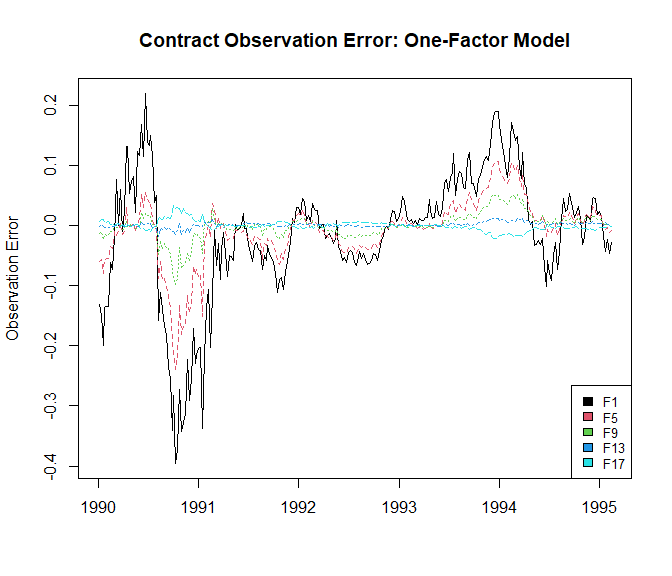##Two-Factor matplot(as.Date(rownames(Oil_2F$V)), Oil_2F$V, type = 'l', xlab = "", ylab = "Observation Error", ylim = c(-0.3, 0.2), main = "Contract Observation Error: Two-Factor Model"); legend("bottomright", colnames(Oil_2F$V),col=seq_len(5),cex=0.8,fill=seq_len(5))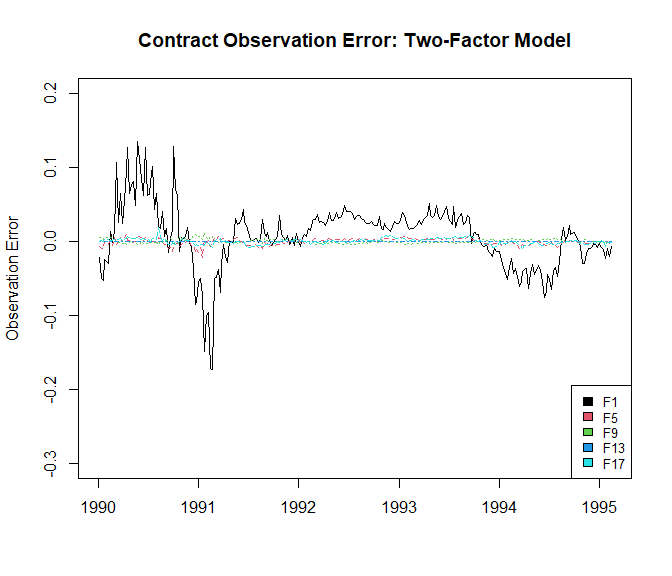Observation errors of the two-factor model were lower than the one-factor model in this case. For the two-factor model, observation error for the one month maturity contract is far higher than the other contracts, meaning the two-factor models ability to predict very short-term contracts is lower than middle and long term contracts.

Comparing this with the contract observation error of the estimated one-factor GBM model:

This is also shown by the root mean squared error (RMSE) of each contract:

matplot(cbind(Oil_1F$Term Structure Fit["RMSE",], Oil_2F$Term Structure Fit["RMSE",]),
type = 'l', main = "Root Mean Squared Error of Futures Contracts",
xlab = "Contract", ylab = "RMSE"); legend("right",c("One-Factor", "Two-Factor"),
col=1:2,cex=0.8,fill=1:2)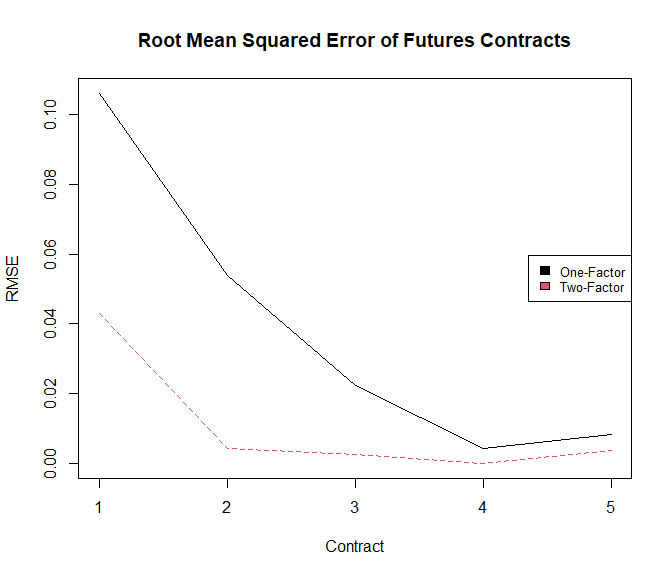The one-factor GBM model had the highest fit for the medium maturity contract, with higher errors for the very short- and long-term contracts, whilst the two-factor model had lower RMSE values overall, and less discrepancy between the RMSE values of the different contracts. These findings are consistent with those found by Aspinall et al. (2020) for the prices of emissions allowances in the European Union.

## 4.8 Plot filtered values:

The two-factor model can be interpreted as the decomposition of spot prices into short-term deviations (factor 2) and long-run equilibrium prices (factor 1). The estimated equilibrium and spot prices were presented in figure 4 of Schwartz and Smith (2000) and are replicated below:

##Replicate Figure 4 of Schwartz and Smith (2000):
SS_figure_4 <- cbind(Equilibrium Price =
exp(Oil_2F$X[,1]), Spot Price = Oil_2F$Y[,"filtered Spot"])

matplot(as.Date(rownames(SS_figure_4)), SS_figure_4, type = 'l',
xlab = "", ylab = "Oil Price ($/bbl, WTI)", col = 1, main = "Estimated Spot and Equilibrium Prices for the Futures Data")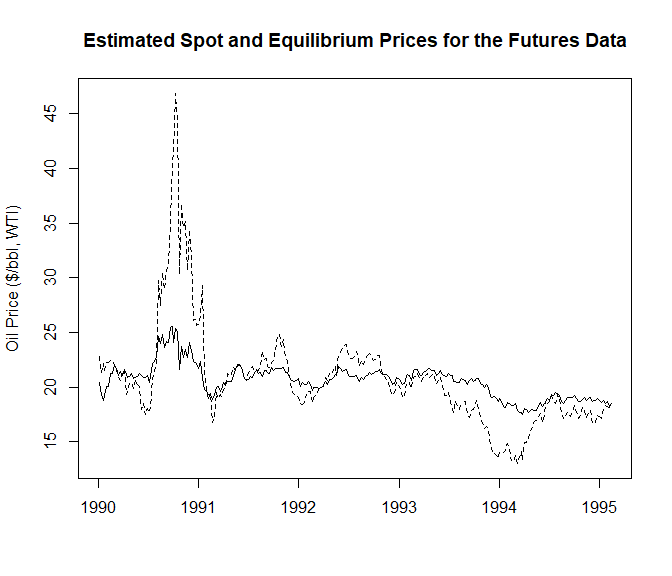## 4.9 Plot Standard Deviation of State Variables: Cortazar and Naranjo (2006) plot the time series of the standard deviation for state variables (Figures 2 & 3) for analysis of the behaviour of the state variables over the observation period. When using stitched, aggregate data (i.e., the time-to-maturity of observations are time-homogeneous) the Kalman filter and covariance of state variables quickly approaches a steady state distribution. Plotting the covariance of state variables of contract data, however, can provide insights and complement analysis into exogenous shocks, structural breaks and the volatility of the factors that influence a commodity over time. When plot(as.Date(rownames(SS_oil$contracts)), sqrt(Oil_2F_contracts$P_t[1,1,]), type = 'l', xlab = "Date", ylab = "Std. Dev.", main = "Time Series of the Std. Dev for State Variable 1")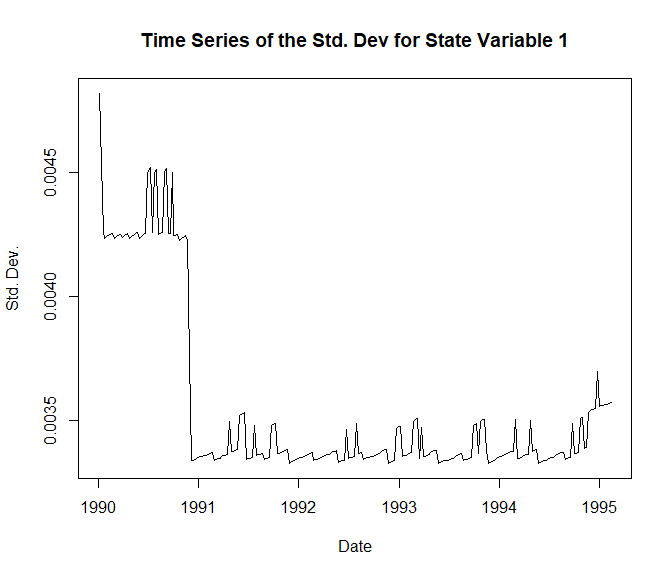A possible structural break at approximately the beginning of 1991 can be observed within the plot above. In the work of Cortazar and Naranjo (2006), structural breaks in time series data were tested by comparing the time-series covariance matrix of state variables for difference samples, and then computing a Wald test statistic. ## 4.10 Forecast Spot and Futures Prices: Expected Spot and futures prices can be forecast under an N-factor model analytically through the ‘spot_price_forecast’ and ‘futures_price_forecast’ functions. ‘help(spot_price_forecast)’ and ‘help(futures_price_forecast)’ presents expressions of expected spot and futures prices respectively. Forecasted spot and futures prices were presented in the work of Schwartz and Smith (2000) in figures 1 and 2 using parameters developed using Enron data. ##Figure 1 and 2 of Schwartz and Smith (2000) was developed using Enron data ##and an assumption that mu was approximately 3% p.a.: Enron_values <- c(0.0300875, 0.0161, 0.014, 1.19, 0.115, 0.158, 0.189) names(Enron_values) <- NFCP_parameters(2, TRUE, FALSE, 0, FALSE, FALSE) ### 4.10.1 Spot Prices: The work of Schwartz and Smith (2000) presented forecasts of spot prices developed using Enron data (figure 1): ## Replicate figure 1 of Schwartz and Smith (2000): SS_expected_spot <- spot_price_forecast(x_0 = c(2.857, 0.119), parameters = Enron_values, t = seq(0,9,1/12), percentiles = c(0.1, 0.9)) ##Factor one only: equilibrium_theta <- Enron_values[!names(Enron_values) %in% c("kappa_2", "lambda_2", "sigma_2", "rho_1_2")] SS_expected_equilibrium <- spot_price_forecast(x_0 = c(2.857, 0), equilibrium_theta, t = seq(0,9,1/12), percentiles = c(0.1, 0.9)) SS_figure_1 <- cbind(SS_expected_spot, SS_expected_equilibrium) matplot(seq(0,9,1/12), SS_figure_1, type = 'l', col = 1, lty = c(rep(1,3), rep(2,3)), xlab = "Time (Years)", ylab = "Spot Price ($/bbl, WTI)",
main = "Probabilistic Forecasts for Spot and Equilibrium Prices")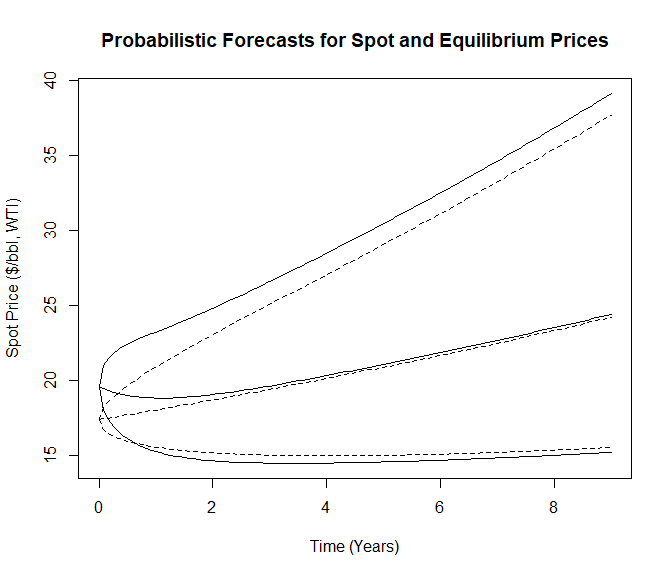An important consideration when forecasting spot prices using parameters estimated through MLE is that the parameter estimation process takes the assumption of risk-neutrality and thus the true process growth rate $$\mu$$ is not estimated with a high level of precision. For example, the standard error of parameter $$\mu$$ for the one-factor GBM estimated in section 4.3 was approximately 10%. This is further shown such that Schwartz and Smith (2000) did not use the estimated value of $$\mu$$ to forecast spot prices, opting to set it at a value “that is more representative of investor expectations” (Schwartz and Smith (2000)).

### 4.10.2 Futures Prices

Risk-neutral parameters are directly observed in the parameter estimation process and are therefore estimated with a higher level of precision.

Futures prices of Enron data was presented in the work of Schwartz and Smith (2000) in figure 2:

## Replicate Figure 2 of Schwartz and Smith (2000):

#Forecast expected spot prices under the "True" stochastic process:
SS_expected_spot <- spot_price_forecast(x_0 = c(2.857, 0.119),
parameters = Enron_values,
t = seq(0,9,1/12),
percentiles = c(0.1, 0.9))
#Forecast expected futures prices under the Risk-Neutral stochastic process:
SS_futures_curve <- futures_price_forecast(x_0 = c(2.857, 0.119),
parameters = Enron_values,
futures_TTM = seq(0,9,1/12))
SS_figure_2 <- cbind(SS_expected_spot[,2], SS_futures_curve)
matplot(seq(0,9,1/12), log(SS_figure_2), type = 'l', col = 1,
xlab = "Time (Years)", ylab = "Log(Price)",
main = "Futures Prices and Expected Spot Prices")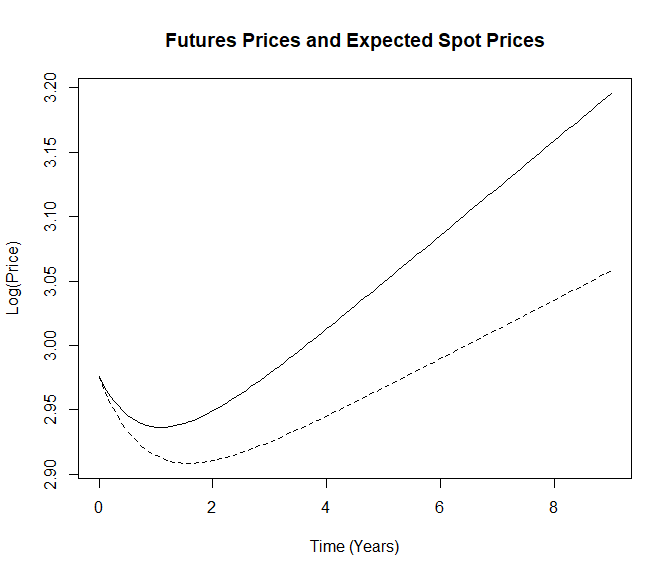### 4.10.3 Plot the Futures Curve:

The model fit to the observed term structure on given dates can be used as a measure of robustness of N-factor commodity pricing models. This also allows for analysis to how the model fits under circumstances of strong backwardation or contango, exogenous shocks, etc.

The futures curve can be forecast on a particular date using the estimate state vector and compared to the observed futures prices at that time. For example, the fit of the estimated one-factor GBM model and the two-factor model of Schwartz and Smith (2000) can be plotted against observed oil futures prices on the final observation date:

## Maximum Observed Maturity:
max_maturity <- max(tail(SS_oil$contract_maturities,1), na.rm = TRUE) ##Estimated Futures Prices: ### One Factor (GBM): oil_TS_1F <- futures_price_forecast(x_0 = Oil_1F$x_t,
parameters = Oil_1F$estimated_parameters, futures_TTM = seq(0,max_maturity,1/12)) ### Two Factor: oil_TS_2F <- futures_price_forecast(x_0 = Oil_2F$x_t,
parameters = SS_oil$two_factor, futures_TTM = seq(0,max_maturity,1/12)) matplot(seq(0,max_maturity,1/12), cbind(oil_TS_1F, oil_TS_2F), type = 'l', xlab = "Maturity (Years)", ylab = "Futures Price ($)",
main = "Estimated and observed oil futures prices on 1995-02-14");
points(tail(SS_oil$contract_maturities,1), tail(SS_oil$contracts,1))
legend("bottomleft", c("One-factor", "Two-Factor", "Observed"),
col=2:4,cex=0.8,fill=c(1,2,0))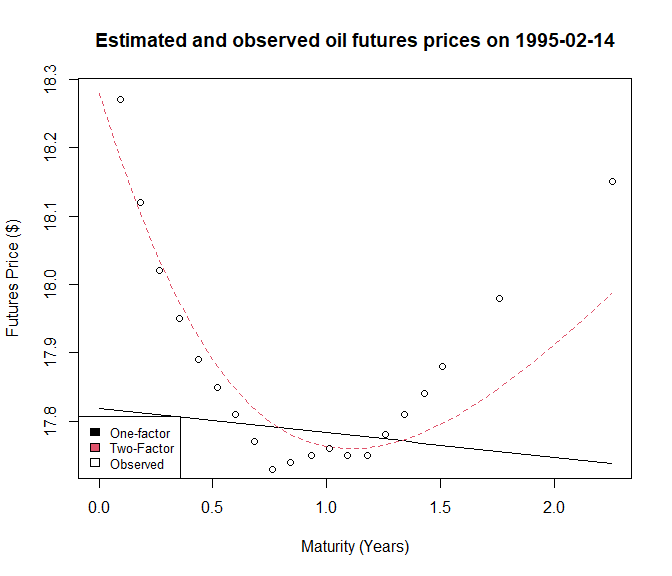The additional flexibility of the two-factor model better fits the observed term structure at this particular observation date, particularly because the one-factor GBM model is linear in forecasts, which does not describe the observed behavior of futures prices. Comparing the models ability across different observation dates and when the market is in different levels of backwardation, contango, etc. can be used as a measure of robustness and fit of commodity pricing models.

The fit of the two-factor model noticeably decreases beyond a maturity of approximately 1.5 years. This is likely due to futures contracts with maturities beyond 17 months not being observed in the two-factor model, due to stitched futures prices being used for parameter estimation. This provides a visual example of the information loss associated with this model due to applying stitched data.

## 4.11 Plot Volatility Term Structure:

In the work of Cortazar and Naranjo (2006), the ability of an N-factor model to match the volatility term structure of futures returns is presented as a measure of robustness (figure 7). This robustness measure can be attained by calling the ‘TSfit_volatility’ function, but it is also returned by the ‘NFCP_Kalman_filter’ function when ‘verbose = TRUE’.

###Test the Volatility Term Structure Fit of the Schwartz-Smith Two-Factor Oil Model:
V_TSFit <- TSfit_volatility(
parameters = SS_oil$two_factor, futures = SS_oil$stitched_futures,
futures_TTM = SS_oil$stitched_TTM, dt = SS_oil$dt)

##Plot Results:
matplot(V_TSFit["Maturity",], cbind(Oil_1F$Term Structure Volatility Fit["Theoretical Volatility",], V_TSFit["Theoretical Volatility",]), type = 'l', xlab = "Maturity (Years)", ylab = "Volatility (%)", ylim = c(0, 0.5), main = "Volatility Term Structure of Futures Returns"); points( V_TSFit["Maturity",], V_TSFit["Empirical Volatility",]); legend("bottomleft", c("Empirical", "One-Factor", "Two-Factor"),col=0:2,cex=0.8,fill=0:2)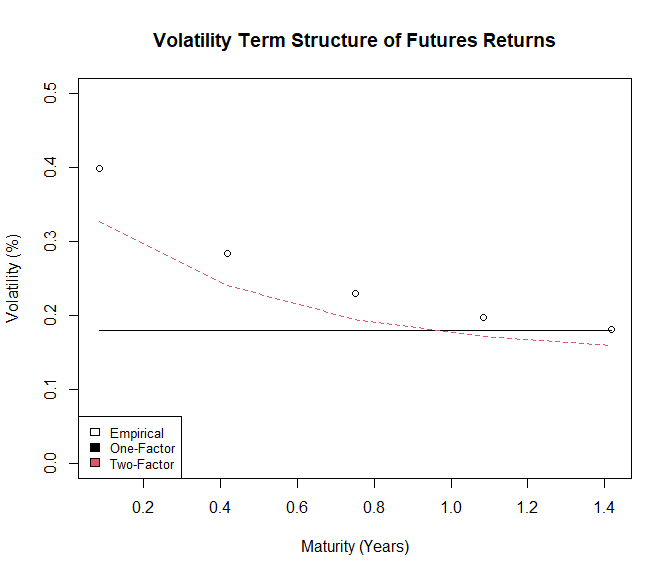This finding shows that the two-factor model tends to slightly underestimate the volatility term structure of futures returns of this dataset. The two-factor model has a better fit to the volatility term structure over the one-factor GBM model, however, as it considers volatility to be a depreciating function with respect to the time to maturity of a futures contract (i.e., the Samuelson’s effect). Additional factors could possibly have a better fit to this robustness measure. A larger number of factors provides the model with more flexibility in adjusting first and second moments simultaneously, possibly explaining the ability for models with more factors to better describe the volatility term structure of futures returns. ## 4.12 Simulate Spot and Futures Prices: Monte Carlo simulation can be used to numerically simulate future price paths of spot and futures prices under an N-factor model through the ‘spot_price_simulate’ and ‘futures_price_simulate’ functions. Valuation of American options under an N-factor model generally requires Monte Carlo methods due to the multidimensional structure of N-factor models. The simulation of future price paths is therefore of great interest to those evaluating American options, investment under commodity price uncertainty, and Real Options Analysis. The solutions of simulating spot and futures prices are presented withing ‘help(spot_price_simulate)’ and ‘help(futures_price_simulate)’. ### 4.12.1 Spot Prices: Spot prices are simulated using the ‘mvrnorm’ function of the ‘MASS’ package to quickly and efficiently estimate futures shocks of price paths. 100 simulations with a forecasting horizon of 1 year and a monthly (ie. 1/12) discrete time step can be computed through the following: ##100 antithetic simulations of one year of monthly observations simulated_spot_prices <- spot_price_simulate( x_0 = Oil_2F$x_t,
parameters = SS_oil$two_factor, t = 1, dt = 1/12, N_simulations = 1e3, antithetic = TRUE, verbose = TRUE) ##Plot Price Paths: matplot(seq(0,1,1/12), simulated_spot_prices$spot_prices, type = 'l',
xlab = "Forecasting Horizon (Years)",
ylab = "Spot Price ($/bbl, WTI)", main = "Simulated Crude Oil prices")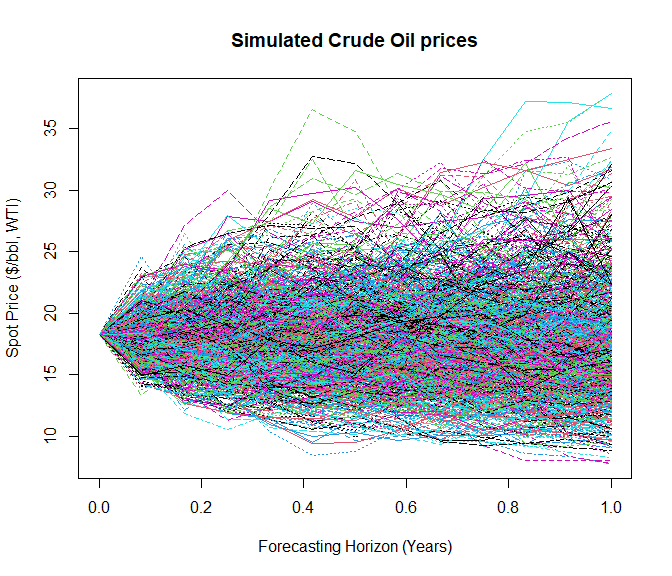Spot prices are simulated using antithetic price paths by default, a simple and common variance reduction technique: Confidence intervals of simulations can also be visualized: ##Plot 95% Prediction interval: prediction_interval <- rbind.data.frame(apply(simulated_spot_prices$spot_prices, 1,
FUN = function(x) stats::quantile(x, probs = c(0.025, 0.975))),
Mean = rowMeans(simulated_spot_prices$spot_prices)) matplot(seq(0,1,1/12), t(prediction_interval), type = 'l', col = c(2,2,1), lwd = 2, lty = c(2,2,1), xlab = "Forecasting Horizon (Years)", ylab = "Spot Price ($/bbl, WTI)", main = "Simulated Crude Oil 95% Confidence Interval")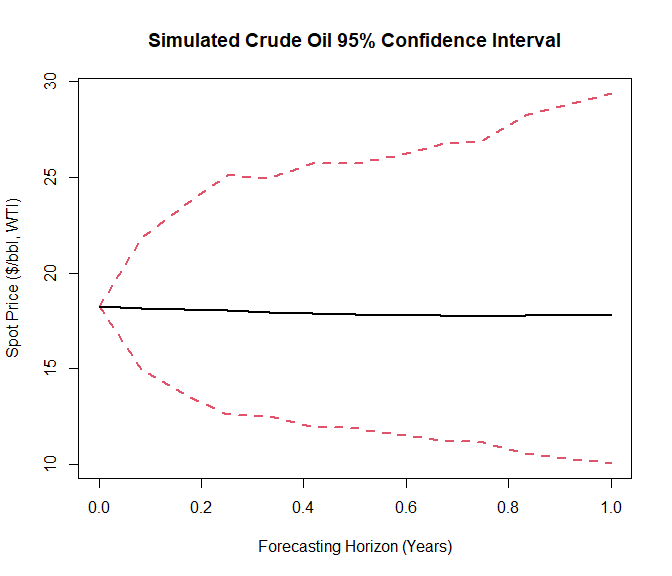### 4.12.2 Futures Prices:

Aggregated, stitched futures data as well as individual contracts are able to be simulated through the ‘futures_price_simulate’ function:

## Simulate Crude Oil Contract Prices under a Two-Factor model

simulated_contracts <- futures_price_simulate(x_0 = c(log(SS_oil$spot[1,1]), 0), parameters = Oil_2F_parameters, dt = SS_oil$dt,
N_obs = nrow(SS_oil$contracts), futures_TTM = SS_oil$contract_maturities)

##Plot Simulated prices:
matplot(as.Date(rownames(SS_oil$contracts)), simulated_contracts$futures_prices,
type = 'l', ylab = "Futures Price ($/bbl, WTI)", xlab = "Observations", main = "Simulated Futures Contracts")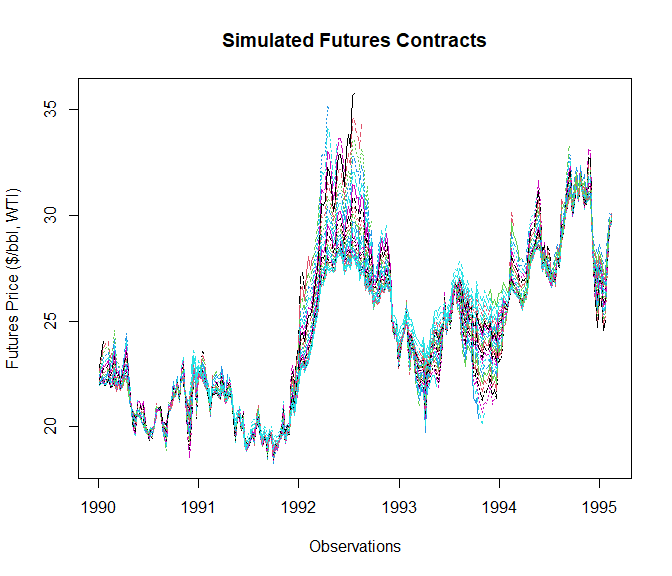Simulating futures prices can be useful for various analytic purposes, such as testing model performance on simulated data where parameter values are known a priori. ## 4.13 Option Valuation: Commodity pricing models can be applied for valuing and hedging commodity contingent claims. The ‘NFCP’ package allows for the pricing of European and American call and put options on futures contracts of commodities through the ‘American_option_value’ and ‘European_option_value’ functions, respectively. Commodity options on futures are the derivative products that give the owner the right, but not the obligation, to buy (call) or sell (put) a particular futures contract. The N-factor framework is a linear Guassian framework of commodity pricing models. As such, there exist analytic expressions for the value of European call and put options for N-factor models. Under the N-factor framework, European call and put options can be valued analytically through the Black and Scholes stock-option model applied to the N-factor framework. Schwartz and Smith (2000) first presented analytic European option pricing under a two-factor model. American options are options with early exercise opportunities for which no closed form solution exists. The least-squares Monte carlo (LSM) simulation option valuation method, first presented by Longstaff and Schwartz (2001), approximates the value of American options. The following section presents the valuation of European and American put options under the N-factor framework, considering and comparing the value of options under both a one-factor and two-factor commodity pricing model. Consider an option with maturity in one year of a futures contract that expires two years from today on oil, with an exercise price of$20/bbl. Further assume the risk-free interest rate is 5% p.a. For the case of American options, consider 100,000 simulations (of which 50% are antithetic) and an exercise opportunity of 50 times per year.

To adequately forecast future prices of oil, we require contemporary estimates of the state variables. Optimal one-step-ahead estimate of state variables can be estimated through the Kalman filter, given that maximum likelihood parameter estimates of the CPM have been calculated. Contemporary state variable estimates are available within the ‘x_t’ object returned by ‘NFCP_Kalman_filter’ and ‘NFCP_MLE’.

The European and American put option prices in this example can be calculated assuming that spot prices follow the one-factor model estimated in section 4.4 and the two-factor model of Schwartz and Smith (2000):

Option_prices <- matrix(rep(0,2), nrow = 2, ncol = 3, dimnames = list(c("One-Factor", "Two-Factor"), c("European", "American", "Early Exercise Value")))

# Strike Price:
strike <- 20
# Annual risk-free interest rate:
risk_free <- 0.05
# Maturity of option and future:
option <- 1
future <- 2
## American option only - number of antithetic simulations, time step (in years):
time_step <- 1/50
monte_carlo <- 1e5

## One-factor European put option value:
Option_prices[1,1] <- European_option_value(x_0 = Oil_1F$x_t, parameters = Oil_1F$estimated_parameters,
futures_maturity = future, option_maturity  = option, K = strike, r = risk_free)
## Two-factor European put option value:
Option_prices[2,1] <- European_option_value(x_0 = Oil_2F$x_t, parameters = SS_oil$two_factor,
futures_maturity = future, option_maturity = option, K = strike, r = risk_free)

## One-factor American put option value:
Option_prices[1,2] <- American_option_value(x_0 = Oil_1F$x_t, parameters = Oil_1F$estimated_parameters,
futures_maturity = future, option_maturity = option, K = strike, r = risk_free,
N_simulations = monte_carlo, dt = time_step)
## Two-factor American put option value:
Option_prices[2,2] <- American_option_value(x_0 = Oil_2F$x_t, parameters = SS_oil$two_factor,
futures_maturity = future, option_maturity = option, K = strike, r = risk_free,
N_simulations = monte_carlo, dt = time_step)

Option_prices[,3] <- Option_prices[,2] - Option_prices[,1]

## Print results:
print(round(Option_prices,3))
#>            European American Early Exercise Value
#> One-Factor    2.628    2.673                0.045
#> Two-Factor    2.399    2.432                0.033

The ability to exercise options at any point before maturity is a premium that American-style options hold over European-style options and therefore the value of American-style options are always greater than, or equal to, European-style counterparts.

The calculated price of European and American options can vary greatly based upon the specified commodity pricing model, providing evidence that the modeling of commodity prices is a crucial consideration of commodity producers and consumers looking to hedge against commodity price uncertainty, as well as other commodity market participants.

When ‘verbose = TRUE’ is set for the ‘European_option_value’ function, the first derivative of different underlying parameters of the N-factor model and European option (i.e., the ‘Greeks’) are returned along with the calculated option price.

When ‘verbose = TRUE’ is set for the ‘American_option_value’ function, simulated characteristics of the option are returned, including the standard error of estimates, the cumulative probability of early exercise over the life of the option and the expected exercise time of the American option.

The ‘American_option_value’ function includes parameter arguments ‘orthogonal’ and ‘degree’. These are arguments relating to the LSM simulation method used to approximate the option value. The convergence properties of the LSM simulation function state that the calculated American option value converges to the true value as the number of simulations and basis functions increase (Longstaff and Schwartz, 2001; Clément, Lamberton and Protter, 2002). It is therefore important to consider that the values ‘N_simulations’ and ‘degree’ are sufficiently high and that ‘dt’ is sufficiently low to allow the ‘American_option_value’ function to adequately converge to the true solution. The convergence of the American option value can be evaluated through the magnitude of the standard error. Different orthogonal polynomials available in the ‘orthogonal’ argument are likely to be insensitive to calculated American option value.

Empirical studies within existing literature has suggested that in order for a commodity pricing model to accurately price European and American financial options, a stochastic volatility specification is required. See Cortazar et al. (2016).

# 5. Discussion:

This vignette has presented the ‘NFCP’ (N-Factor Commodity Pricing) package, providing worked examples of the functionality of the package, replicating the work of Schwartz and Smith (2000) throughout the study. The ‘NFCP’ package is a very versatile package that provides the ability to effectively build, analyse, forecast, simulate and value the derivate products of commodity prices. The ‘NFCP’ package allows for a wide versatility in the structure of commodity pricing models, allowing the spot price process of the commodity to be made up of the exponential sum of N arbitrary factors, with both random walk and mean-reverting characteristics available, as well as a deterministic seasonality component. The ‘NFCP’ package combines the fastest Kalman filter algorithm available with an effective optimization algorithm to ensure MLE returns optimal parameters for a given commodity pricing model and term structure data.

The numeric difficulty of parameter estimation increases sharply with the number of underlying factors, N. The number of unknown parameters of an N-factor model with M measurement errors is equal to: $$0.5 (N^2 + 5N) + M$$. Realistically, commodity pricing models with more than four or five factors are impractical due to the difficulty of parameter estimation through MLE.

The parameter estimation process is a bounded search under which the parameters can only take realistic values (i.e. volatility terms cannot be negative, etc.). Parameters estimated through MLE, however, have no in-built guarantee that they will make explicit economic sense. Output analysis of MLE is a crucial step in ensuring commodity pricing models are appropriate for analysis purposes. Factors with extremely large estimated correlation coefficients (say, greater than 90% or less than -90%) are generally poorly specified, with the commodity pricing model failing to differentiate between the dynamics that drive these two factors. Mean-reverting factors with extremely large risk-premium parameters ,$$\lambda_i$$, are considered poorly specified models and generally include factors with very high correlation coefficients. Finally, reversion rates, $$\kappa_i$$, that are extremely high or low are a final indicator of poorly specified models. A reversion rate that is too low is representative of a factor that does not revert over time, thus inducing a unit root in the spot price process. If this is encountered when GBM = FALSE, this may be the model naturally inducing a unit root, indicating that a degree of the spot price process is driven by a random walk. If this is encountered when GBM = TRUE, it’s not appropriate to utilize the commodity pricing model under the assumption that spot prices are driven by more than one unit-root process. When mean-reversion rates are extremely high, the state variable approaches a deterministic value given by the equilibrium of the process. Although log-likelihood scores for some commodity pricing models may indicate their increased ability to explain the term structure of a commodity, output analysis of parameters estimated through MLE is a crucial step to evaluating the applicability of estimated models for forecasting and analysis purposes.

Finally, estimated commodity pricing models can be used to value commodity contingent claims, such as capital investment decisions under commodity price uncertainty. Estimated commodity pricing models are ideal for the input into real options frameworks to value investment projects where futures cost or revenue cash flows are dependent upon future prices paths of commodities. LSM simulation can be used to value and optimize investment decisions of these capital investment opportunities. This involves the simulation of the the spot price of the commodity, calculating payoffs at each time point contingent on simulated commodity prices. The ‘LSMRealOptions’ package provides a framework for the calculation of American-style option values, including the valuation of capital investment projects, through the LSM simulation method (Aspinall et at., 2021). ‘LSMRealOptions’ natively supports the simulated factors output by the ‘spot_price_simulate’ and is appropriate for other derivative pricing of commodity contingent claims.

The ‘NFCP’ package is a comprehensive and powerful framework for the development and analysis of commodity pricing models. It provides the ability to forecast and value derivative products using existing term structure data, and further provides the tools required to value American option products through simulation methods. The development and analysis of commodity pricing models is highly recommended to those who interact and trade in commodity markets, or those who have investment projects with the returns of these project contingent on the prices of commodities.